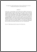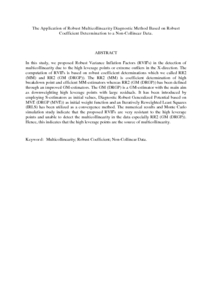# The Application of Robust Multicollinearity Diagnostic Method Based on Robust Coefficient Determination to a Non-Collinear Data.

## Citation

Midi, Habshah and Bagheri, Arezoo and Imon, A.H.M Rahmatullah (2010) The Application of Robust Multicollinearity Diagnostic Method Based on Robust Coefficient Determination to a Non-Collinear Data. Journal of Applied Sciences, 10 (8). pp. 611-619. ISSN 1812-5654

## Abstract

In this study, we proposed Robust Variance Inflation Factors (RVIFs) in the detection of multicollinearity due to the high leverage points or extreme outliers in the X-direction. The computation of RVIFs is based on robust coefficient determinations which we called RR2 (MM) and RR2 (GM (DRGP)). The RR2 (MM) is coefficient determination of high breakdown point and efficient MM-estimators whereas RR2 (GM (DRGP)) has been defined through an improved GM-estimators. The GM (DRGP) is a GM-estimator with the main aim as downweighting high leverage points with large residuals. It has been introduced by employing S-estimators as initial values, Diagnostic Robust Generalized Potential based on MVE (DRGP (MVE)) as initial weight function and an Iteratively Reweighted Least Squares (IRLS) has been utilized as a convergence method. The numerical results and Monte Carlo simulation study indicate that the proposed RVIFs are very resistant to the high leverage points and unable to detect the multicollinearity in the data especially RR2 (GM (DRGP)). Hence, this indicates that the high leverage points are the source of multicollinearity.Preview
PDF (Abstract)
The Application of Robust Multicollinearity Diagnostic Method Based on Robust Coefficient Determination to a Non.pdfView Item